# 统计学①——概率论基础及业务实战

## 二、概率论基础

1、概率是什么？

2、各种事件？

3、何为交集并集？
A∩B： A和B的交集，P(A∩B)代表A和B同时发生的概率

A∪B: A和B的并集，P(A∪B)代表A和B任意一个发生的概率

3、条件概率是个啥？

P(B|A) = P(A∩B)/P(A)

4、全概率公式是？

P(B）= P(B|A)*P(A) + P(B|A’)*P(A’)
A’ 是指A不发生

5、贝叶斯定理好像有很名？

P(A∩B) = P(A)*P(B|A) = P(B)*P(A|B)
P(B）= P(B|A)*P(A) + P(B|A’)*P(A’)

P(A|B) = P(B|A)*P(A) / P(B|A)*P(A) + P(B|A’)*P(A’)

## 三、随机变量

P(X = x1)就是指X为x1的概率

1、随机变量期望如何算？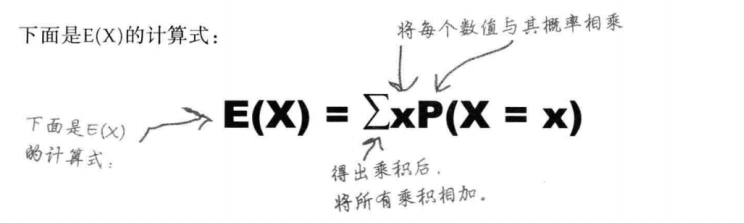2、随机变量方差如何算？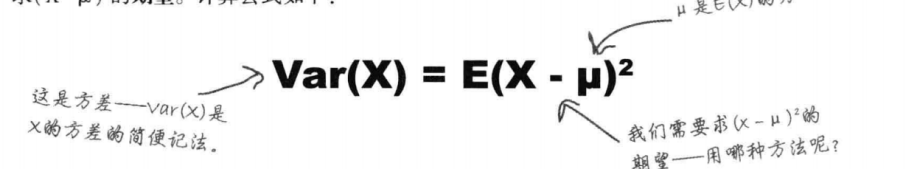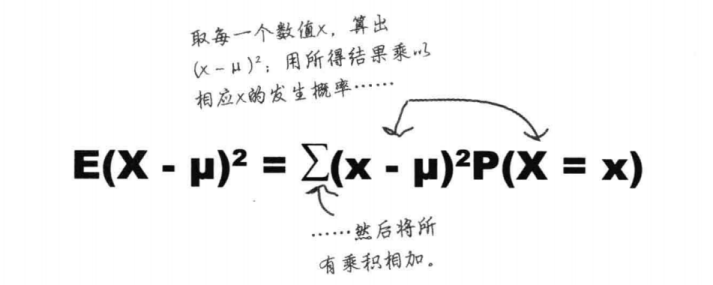3、如果随机变量的取值做了线性变换，怎么计算？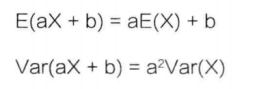4、如果多个独立的随机变量一起，要如何计算呢？

E(X + Y ) = E(X) + E(Y)
Var(X+ Y ) = Var(X) + Var( Y)

5、一个随机变量重复多次，怎么计算呢？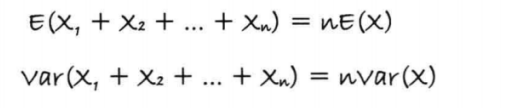## 五、 业务实战

E(X) = ∑ x*P(X=x) = 9.55
max Var(X) = E(X-μ)^2 = ∑(x-μ)^2*P(X=x)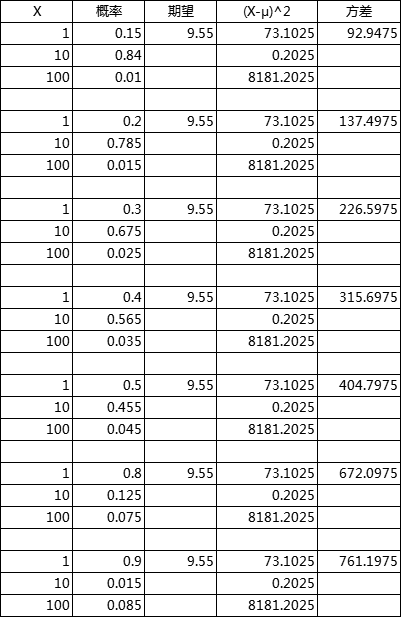03-262113
08-084449

04-217560
05-312110
04-196319
02-292020
12-191072
06-303428
07-182万+
07-212174
07-164849
07-162246
08-16681
06-30412
08-286938
06-142万+
05-211万+
08-1980
03-20407

### “相关推荐”对你有帮助么？

•非常没帮助
•没帮助
•一般
•有帮助
•非常有帮助¥2 ¥4 ¥6 ¥10 ¥20余额支付 (余额：-- )扫码支付获取中扫码支付点击重新获取扫码支付1.余额是钱包充值的虚拟货币，按照1:1的比例进行支付金额的抵扣。
2.余额无法直接购买下载，可以购买VIP、C币套餐、付费专栏及课程。余额充值